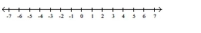# Precalculus Graphical Numerical Algebraic

Mathematics

## Quiz 12 : PrerequisitesLooking for Precalculus Homework Help?

## Quiz 12 :Prerequisites

Question TypeFind the decimal form for the rational number. If it repeats, then so indicate with a bar. - $\frac { 9 } { 2 }$
Free
Multiple Choice

C

TagsFind the decimal form for the rational number. If it repeats, then so indicate with a bar. - $\frac { 17 } { 2 }$
Free
Multiple Choice

B

TagsFind the decimal form for the rational number. If it repeats, then so indicate with a bar. - $- \frac { 22 } { 3 }$
Free
Multiple Choice

A

TagsFind the decimal form for the rational number. If it repeats, then so indicate with a bar. - $\frac { 14 } { 9 }$
Multiple Choice
TagsFind the decimal form for the rational number. If it repeats, then so indicate with a bar. - $\frac { 11 } { 4 }$
Multiple Choice
TagsFind the decimal form for the rational number. If it repeats, then so indicate with a bar. - $\frac { 19 } { 4 }$
Multiple Choice
TagsFind the decimal form for the rational number. If it repeats, then so indicate with a bar. - $\frac { 10 } { 6 }$
Multiple Choice
TagsFind the decimal form for the rational number. If it repeats, then so indicate with a bar. - $- \frac { 29 } { 6 }$
Multiple Choice
TagsFind the decimal form for the rational number. If it repeats, then so indicate with a bar. - $- \frac { 3 } { 8 }$
Multiple Choice
TagsFind the decimal form for the rational number. If it repeats, then so indicate with a bar. - $\frac { 2 } { 5 }$
Multiple Choice
TagsDescribe and graph the interval of real numbers. - $( 3,7 ]$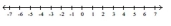Essay
TagsDescribe and graph the interval of real numbers. - $( 7 , \infty )$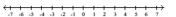Essay
TagsDescribe and graph the interval of real numbers. - $[ - 4,1 ]$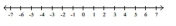Essay
TagsDescribe and graph the interval of real numbers. - $[ - 2,3 )$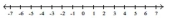Essay
TagsDescribe and graph the interval of real numbers. - $( - 2,3 )$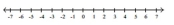Essay
TagsDescribe and graph the interval of real numbers. - $( - \infty , 3 ]$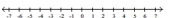Essay
TagsDescribe and graph the interval of real numbers. - $x \leq 4$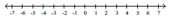Essay
TagsDescribe and graph the interval of real numbers. - $- 3 < x \leq 4$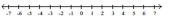EssayDescribe and graph the interval of real numbers. - $x \text { is positive }$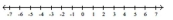Describe and graph the interval of real numbers. - $x \text { is greater than } - 4 \text { and less than or equal to } 2 \text {. }$